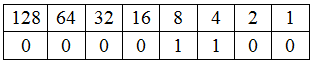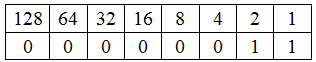## C - Operators

Operators:
Operators are symbols and used to perform some mathematics operations.
There are 6 kinds of operators,
1.Arithmetic operators:
This is normal mathematic operations such as,

Example program for the arithmetic operator:

### #include<stdio.h>  #include <conio.h>  void main()  {     int a,b;     clrscr();     printf("Enter a and b value:");     scanf("%d %d",&a,&b);     printf("Addition value is:%d\n",a+b);     printf("Subtraction value is:%d\n",a-b);     printf("Multiplication value is:%d\n",a*b);     printf("Division value is:%d\n",a/b);     printf("Modulo value is:%d\n",a%b);     printf("a++ value is:%d\n",a++);     printf("b-- value is:%d\n",a--);    getch(); }

Output:

2. Relational Operators:
It is used to compare two or more data.

Note:
This logical operators mostly used in conditions.

3. Logical Operators:
It is used to combine simple relational statements into more complex expressions.

4. Assignment Operators:
This operators are used to getting the result in the same variable(input/output).

Example program for the assignment operator:

### #include<stdio.h>  #include <conio.h>  void main()  {     int a;     clrscr();     printf("Enter a  value:");     scanf("%d",&a);     a+=20;     printf("a value is:%d",a);     getch(); }

Output:

5. Conditional Operators (? :)
It checks itself the condition and execute the statement depending on the condition.

Syntax:

Condition?exp1:exp2;

Example program for the conditional operator:

### #include<stdio.h>  #include <conio.h>  void main()   {      int a=5,b=3,big;    clrscr();  big=a>b?a:b;  printf("Biggest value is %d ",big);  getch(); }

Output:

6. Bitwise Operators:
It is used to manipulate the data at a bit level and it operates on integer only.
Binary table

Bitwise AND(&):
The truth table for ‘ & ‘is shown below(It means True=1 and false=0.)

a=7 of binary code:0111
b=8 of the binary code is:1000
The result of the a&a value is 0000(i.e 0).

Example program for the bitwise AND operator:

### #include <stdio.h> #include<conio.h>  void main() {                    unsigned int a;                    unsigned int b;                    int c = 0;                             clrscr();                    printf("Enter a and b value : ");                    scanf("%d %d",&a,&b);                    c = a&b;                        printf("Bitwise AND of a&b value is: %d\n", c );                    getch();  }

Output:

Bitwise OR(|):
The truth table for ‘ | ‘is shown below

a=7 of binary code:0111
b=8 of the binary code is:1000
The result of a|b value is 1111(i.e 15).

Example program for the bitwise OR operator:

### #include <stdio.h> #include<conio.h> void main() {                    unsigned int a;                    unsigned int b;                    int c = 0;                             clrscr();                    printf("Enter a and b value : ");                    scanf("%d %d",&a,&b);                    c =a|b;                        printf("Bitwise OR of a|b value is: %d\n", c );                    getch(); }

Output:

Bitwise Exclusive OR(^):
The truth table for ‘ ^ ‘is shown below

a=13 of binary code:1101
b=8 of the binary code is:1000
The result of a^b value is 0101(i.e 5).

Example program for the bitwise Exclusive OR operator:

### #include <stdio.h> #include<conio.h> void main() {                    unsigned int a;                    unsigned int b;                    int c = 0;                             clrscr();                    printf("Enter a and b value : ");                    scanf("%d %d",&a,&b);                    c =a^b;                        printf("Bitwise Exclusive OR of a^b value is: %d\n", c );                    getch(); }

Output:

Bitwise Shift left (<<):
Each box called bits and a=6 and a<<1 then a=12.
Explanation:

Result: a of left shit value is 12.Example program for the bitwise shift left operator:

### #include <stdio.h> #include<conio.h> void main() {                     unsigned int a;                    int c = 0;                              clrscr();                    printf("Enter a  value : ");                    scanf("%d",&a);                    c =a<<1;                        printf("Bitwise left shit of a value is: %d\n", c );                    getch(); }

Output:

Bitwise Shift right(>>):
The shit right is similar to the left shit.
For example, If a=6 and a>>2 then its value is 3.

Explanation:

Result: a of right shit value is 3.Example program for the bitwise shift right operator:

### #include <stdio.h> #include<conio.h> void main() {                    unsigned int a;                     int c = 0;                              clrscr();                    printf("Enter a value : ");                    scanf("%d",&a);                    c =a>>1;                        printf("Bitwise right shit of a value is: %d\n", c );                    getch(); }

Output:

Bitwise One's complement(~):
The truth table for ‘ ~ ‘is shown below

If a=6(binary code is 0110),
and ~a(0111) value is 7.
using a negative signature for one's complement(~a=-7).

Example program for the bitwise one's complement operator:

Output: